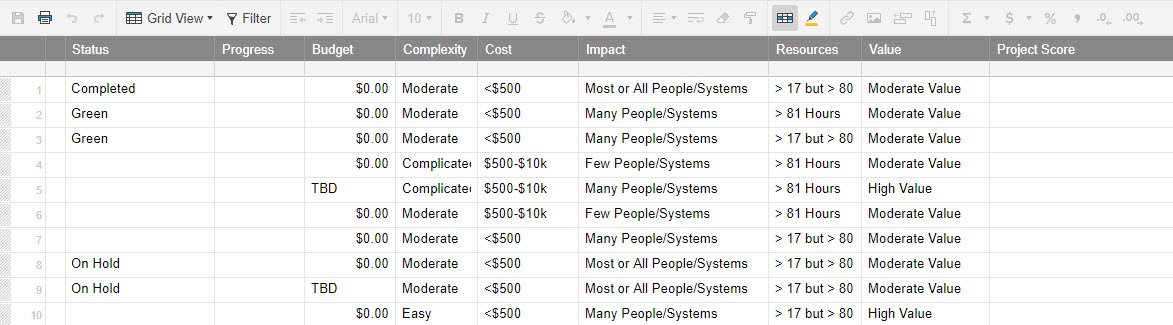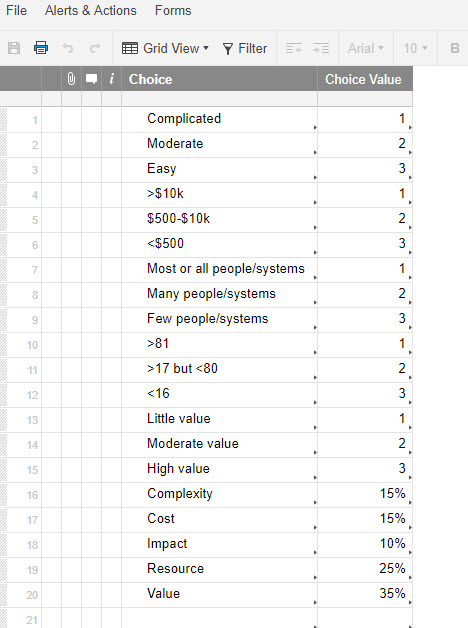or Explore Discussions

# Formula Assistance

07/26/18 Edited 12/09/19

I am attempting to automate my project list by scoring each project based on calculations in another sheet.

I would like to create a "Project Score"  based on drop-downs in my project sheet.   Sum=Complexity*weight + cost*weight + impact*weight +resources*weight + value*weight.   The values of each category and weight are in another sheet.  (pics attached for both sheets to reference).

Any assistance would be greatly appreciated.

J• For this I will call the sheet where you are putting the formula "Target Sheet", and the sheet where your reference table is will be call "Reference Sheet".

This can be done using a series of VLOOKUPs within your SUM function.

We will also use the "Reference Another Sheet" option in the Formula Help Box (see below). Whenever you see {Reference Sheet Range 1}, that is where you will use this. To set this range, click on the "Reference Another Sheet" link when in the appropriate portion of the formula. You will then select both column of data in your Reference Sheet.

So give this a shot. All you should have to change from the below formula is where it says "Weight", simply enter the value you want each field weighted by...

=SUM(VLOOKUP([email protected], {Reference Sheet Range 1}, 2, false) * Weight, VLOOKUP([email protected], {Reference Sheet Range 1}, 2, false) * Weight, VLOOKUP([email protected], {Reference Sheet Range 1}, 2, false) * Weight, VLOOKUP([email protected], {Reference Sheet Range 1}, 2, false) * Weight, VLOOKUP([email protected], {Reference Sheet Range 1}, 2, false) * Weight)

thinkspi.com

• Paul-

Man, I can't thank you enough.  Bonehead moment, all along I was not putting the SUM and going straight for the vlookup.  2 full days on this!  Thank you for saving my sanity, everything worked perfect.

J

• No worries. I've had more than a couple of bonehead moments this week.

Glad we could get it figured out for you though.

thinkspi.com